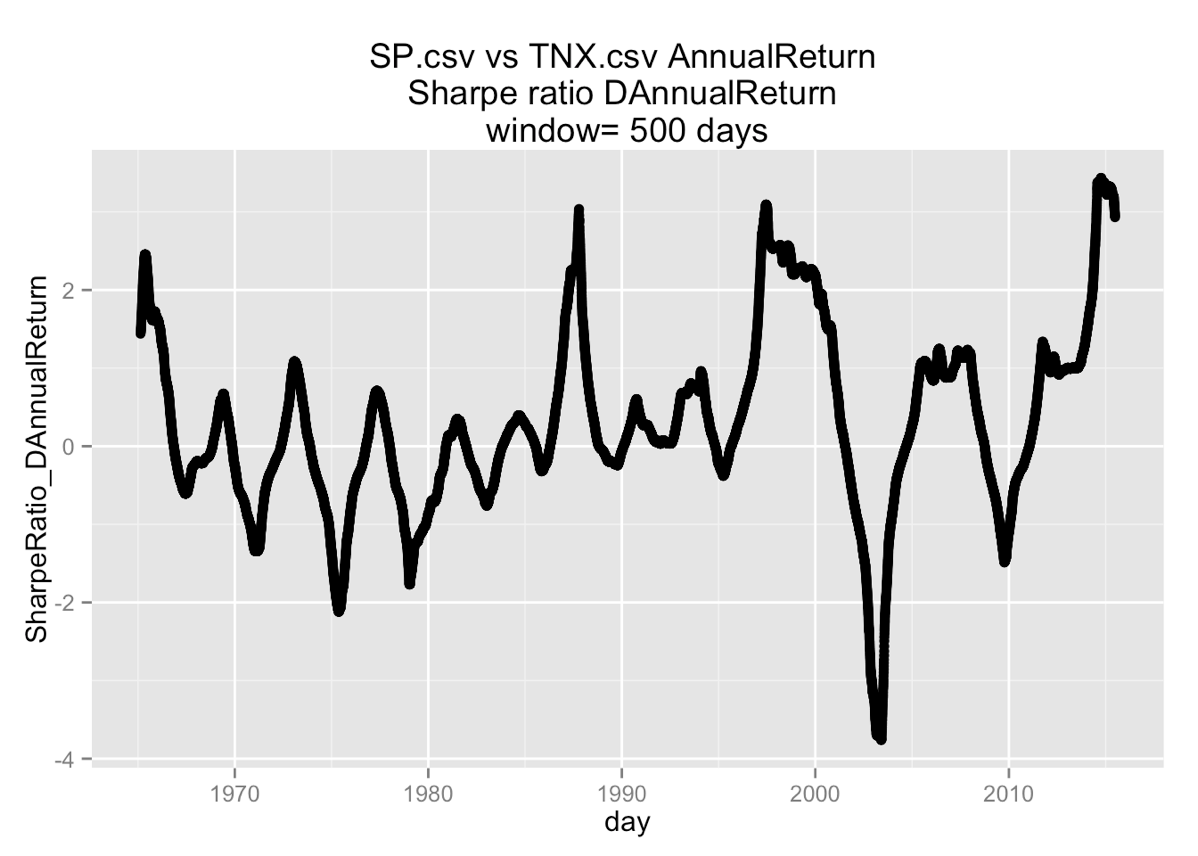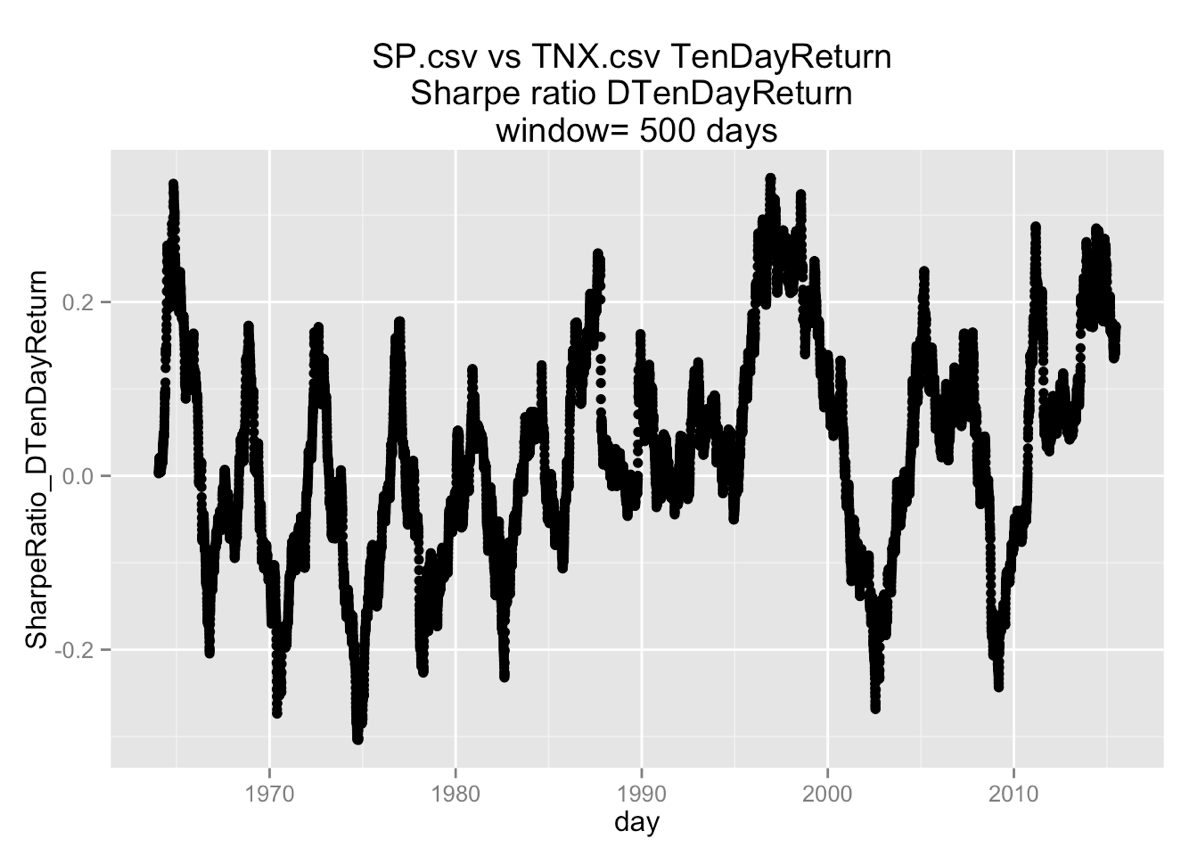# What is a good Sharpe ratio?

We have previously written that we like the investment performance summary called the Sharpe ratio (though it does have some limits).

What the Sharpe ratio does is: give you a dimensionless score to compare similar investments that may vary both in riskiness and returns without needing to know the investor’s risk tolerance. It does this by separating the task of valuing an investment (which can be made independent of the investor’s risk tolerance) from the task of allocating/valuing a portfolio (which must depend on the investor’s preferences).

But what we have noticed is nobody is willing to honestly say what a good value for this number is. We will use the R analysis suite and Yahoo finance data to produce some example real Sharpe ratios here so you can get a qualitative sense of the metric.

“What is a good Sharpe ratio” was a fairly popular query in our search log (until search engines stopped sharing the incoming queries with mere bloggers such as myself). When you do such a search you see advice of the form:

… a ratio of 1 or better is considered good, 2 and better is very good, and 3 and better is considered excellent …

Some sources of this statement include:

Reading these together you see a bit of a content-free echo chamber. Remember: on the web when you see the exact same answer again and again it is more likely due to copying than due to authoritativeness. The last reference indicates a part of the problem: once somebody claims some specific number (such as 1) is a middling Sharpe ratio, no-one dares call any smaller number good (for fear of looking weak).

One also wonders of “2 is good” is some sort of confounding interpretation of the Sharpe ratio as a Fisher style Z statistic (which uses the same ratio of mean over deviance). The point being the rule of thumb “two standard deviations has a two-sided significance of 0.0455” falls fairly close to the heavily ritualized p-value of 0.05.

The correct perspectives about Sharpe ratio are a bit more nuanced:

• Morningstar classroom: How to Use the Sharpe Ratio

Of course, the higher the Sharpe ratio the better. But given no other information, you can’t tell whether a Sharpe ratio of 1.5 is good or bad. Only when you compare one fund’s Sharpe ratio with that of another fund (or group of funds) do you get a feel for its risk-adjusted return relative to other funds.

In fact it is Morningstar that gave a specific range for annual returns (around 0.3) that I used in my article Betting with their money (though now I am not sure I have enough context to be sure if the number they gave was a real example or just notional).

The theory of the Sharpe ratio is: if you have access to the ability to borrow or lend money, then for two similar investments you should always prefer the one with higher Sharpe ratio. So the Sharpe ratio is definitely used for comparison. When I was in finance I used the Sharpe ratio for comparison, but I didn’t have a Sharpe ratio goal.

Reading from a primary source we see estimating the Sharpe ratio of a particular investment at a particular time depends on at least the choice of:

• Investment time frame: are we talking about daily, monthly, quarterly, or annual returns? Changing scale changes returns (daily returns compound about 365 times to get yearly returns!) and changes deviation (theoretically daily returns tend to have a deviation that is around `sqrt(365)` time more volatile than yearly returns). The Sharpe ratio is the ratio of these two quantities, and they are not varying in similar ways as we change scale.
• Choice of “risk free” reference returns. “Return” in the Sharpe ratio is actually defined as “excess return over a chosen risk-free investment.” Choose a comparison investment with low returns and you artificially look good.
• Length of data used to estimate empirical variance (as we are talking about Ex Post Sharpe Ratio, which means we don’t have a theoretical variance to use). In theory (for normal data or data with bounded theoretical variance) variance/deviation estimates should stabilize at moderate window sizes. Picking a too small window may let you avoid some rare losses and display an elevated Sharpe ratio. Picking a too large window may let in data from markets climates not relevant to the current market.

So without holding at least these three choices constant, it doesn’t make a lot of sense to compare.

We emphasize because the Sharpe ratio itself varies over time (even with the above windowing) it in fact does not strictly make sense to talk about “the Sharpe ratio of an investment.” Instead you must consider “the Sharpe ratio of an investment at a particular time” or “the distribution of the Sharpe ratio of an investment over a particular time interval.” This means if you want to estimate a Sharpe ratio for an investment you at least must specify an additional time scale or smoothing window to average over.

For example: below is the Sharpe ratio of the S&P500 index annual returns using a 500 day window to estimate variance/deviation using the 10-year US T-note interest rate as the risk-free rate of return (note we are using the interest rate as the risk-free return, we are not using the returns you would see from buying and selling T-notes). Note: the choice of “risk free” investment here is a bit heterodox.Notice two things:

• The ratio is all over the map, we can call the S&P Sharpe ratio just about any value between -2 and 2 by picking the right 5 year period to consider “typical.” The mean over the period graphed (1960 through 2015) is 0.3 and the median is 0.17.
• The ratio spends a lot of time well below 1 over this history.

For our betting article we needed a Sharpe ratio on a 10 day scale. Here is the S&P500 index 10 day returns using a 500 day window to estimate variance/deviation using the 10-year US T-note interest rate as the risk-free rate of return:Over the graphed time interval viewed we have the upper quartile value is 0.1. So the S&P 10 day return Sharpe ratio spends 75% of its time below 0.1. Thus the 10 theoretical day Sharpe ration of 0.18 in “Betting with their money” is in fact large. Though we have found this calculation is sensitive to the length of the window used to estimate variance (for example using a window of 30 days gives us mean: 0.08, median: 0.075, 3rd quartile 0.45).

And for fun here is a similar Sharpe ratio calculation for the PIMCO TENZ bond fund:This just confirms the last few years have not been good for US bonds.

Note: it is traditional to use very low interest rate instruments as the “safe comparison” in Sharpe ratio. So using 10 T-note interest rates gives an analysis that is a bit pessimistic (and also ascribes the T-note variance to the instrument being scored). However, the “safe comparison” is really only used in the Sharpe portfolio argument as the rate you can borrow and/or lend money at (which is not in fact risk-free in the real world). So there is some value in using an easy to obtain realistic “boring investment” as a proxy for the “risk-free return rate.” The ignoring of the risk-free rate in the Betting with their money article is also not strictly correct (but also something Sharpe ignored for a while), but given the scale of potential wins and losses in that set-up it is not going to cause significant issues.

Basically remember this: there are a lot of analyst chosen details in estimating a Sharpe ratio. One of the biggest ones you can fudge is the estimate of deviation/variance (be it theoretical/Ex-Ante or Ex-Post). I would say very high Sharpe ratios or more likely evidence of under estimating the deviation/variance and the reference return process than evidence of actual astronomical risk-adjusted returns.

The complete R code to produce these graphs from downloaded finance data is given here.

Categories: Finance Mathematics Opinion Tutorials

Tagged as:### jmount

Data Scientist and trainer at Win Vector LLC. One of the authors of Practical Data Science with R.

### 6 replies ›

1.jmount says:
2.Highgamma says:

Nice post. I enjoyed it. I would make one correction. The adjustment from daily to annual Sharpe ratio is by the square root of 252 (or so), the number of trading days in a year. You are calculating the standard deviation using only trading days data (not days for which the market is closed), so you use the number I’d trading days in the year for the adjustment. (Think of it as sampling from a continuous time process. )

1.jmount says:

Highgamma, thanks you are right: the theoretical standard deviation correction should be number of actual trading days. And the compounding of rates remands date based. Fortunately I never used these empirical transforms in the article (I calculated returns for each time period by lagging, which has its own issues).

3.Francesco Ceci says:

Hi John, thanks for sharing your thoughts on the Sharpe ratio. I just wanted to add that besides the choice of the scale of returns (daily, monthly…) , the risk free rate and the length of data used, even a simple transformation from discrete returns to log returns, often used for their easier algebra, affects the Sharpe ratio (i.e. the Sharpe ratio computed on the log returns of a price is usually quite different from the Sharpe ratio on the discrete returns of the same price…). Did Bill Sharpe ever clarify if the mean and the std dev of the returns are meant to be computed on discrete or log returns?

So far, from a practical stanpoint it seems to be that a reasonable suggestion is to compare risk and returns across different dimensions and using several different measures and ratios (although each of them has its own drawbacks). However I would definitely appreciate if somebody comes up with a better idea!

1.jmount says:

Francesco, I agree. I wish just one more step in the calculation was shown (the formation of returns from close prices) to confirm what is meant by return. I know log-returns are a legit metric, but I am pretty sure the standard Sharpe ratio calculation depends on returns that are of the form (1- finalPrice/initialPrice). The point being it is convenient to have no effect be a zero return (not a 1) and the borrowing/loaning portfolio arguments that justify the Sharpe ratio assume you are working in money units (not log-money units).

In the R code I tried some games (putting zero as the risk-free comparable). I also thought about (but didn’t bother with after seeing the zero result) computing the variance only on the instrument (and not on the instrument minus the risk free reference). But it looks there is a lot of sensitivity to what sort of window you are estimating variance (in particular neglect of serial correlation).

4.jmount says:

All specific questions of how things were calculated and how the graphs are made are answered in the “.Rmd” file in the Github archive ( https://github.com/JohnMount/Sharpe ). I also added examples of using zero or the Fed prime-rate as the rate of risk-free return.